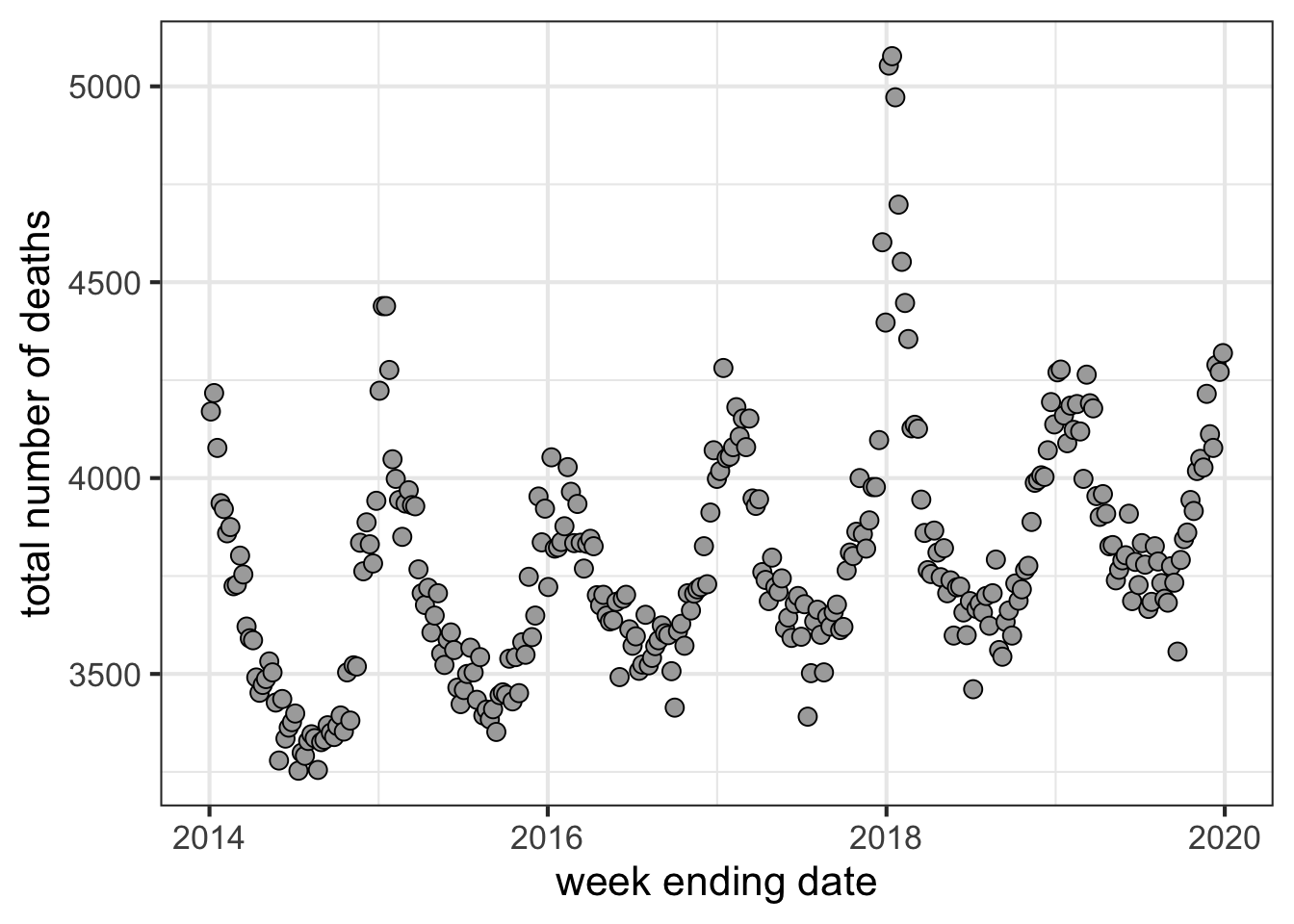The plan is to model the excess mortality from the February winter storms in Texas. I saw the BuzzFeed analysis and article. I thought it might be beneficial to implement a more modern time series modeling approach and to make a comparison to large states rather than small neighboring states. Below is what came of it.

Spoiler: my conclusion is similar to that of BuzzFeed though I think the number is probably not as high as the 700 that BuzzFeed estimated. Ultimately, it is hard to tell exactly since there is noise due to random deviations from model expectations and those deviations in large states often amount to 250 deaths weekly in similarly sized states. Thus, the 750 deaths in excess of the model exceeded random expected deviation, but it may only be 500 deaths or so greater than expected. Indeed Florida and California saw deviations in excess of 400 deaths and California had several weeks with greater than 500 deaths above the model.

I obtained the data directly from CDC in the manner described by BuzzFeed so it would be completely independent of the BuzzFeed analysis.

## Let’s import the data

First, I import the data. Then, I perform some overly complicated manipulations to combine New York and New York City so it can be incorporated in the analysis as a full state. I’m sure there is a more parsimonious way to accomplish this task, but I just wanted to finish the analysis. The data that I call training data is the the pre-2020 data and the test data is the data starting from January 1st, 2020. At the end of the below code chunk, I convert it to a time series object to facilitate further analyses.

``````training.df <- read_csv('data/mortality_data_pre2020.csv')

training.df <- training.df %>%
clean_names() %>%
select(-starts_with('flag')) %>%
mutate(week_ending_date = mdy(week_ending_date)) %>%
filter(jurisdiction_of_occurrence != 'United States') %>%
mutate(across(where(is.character), str_remove_all, pattern = fixed(" ")))

tmp.df <- bind_cols((training.df %>% filter(jurisdiction_of_occurrence == 'NewYork') %>% select(colnames(training.df)[1:4])), (training.df %>% filter(jurisdiction_of_occurrence == 'NewYork') %>% select(colnames(training.df)[5:ncol(training.df)])) + (training.df %>% filter(jurisdiction_of_occurrence == 'NewYorkCity') %>% select(colnames(training.df)[5:ncol(training.df)])))

training.df <- training.df %>%
filter(jurisdiction_of_occurrence != 'NewYork' &
jurisdiction_of_occurrence != 'NewYorkCity') %>%
bind_rows(tmp.df) %>%
arrange(jurisdiction_of_occurrence, week_ending_date)
rm(tmp.df)

test.df <- test.df %>%
clean_names() %>%
select(-starts_with('flag')) %>%
mutate(week_ending_date = ymd(week_ending_date)) %>%
filter(jurisdiction_of_occurrence != 'United States') %>%
mutate(across(where(is.character), str_remove_all, pattern = fixed(" ")))

tmp.df <- bind_cols((test.df %>% filter(jurisdiction_of_occurrence == 'NewYork') %>% select(colnames(test.df)[1:4])), (test.df %>% filter(jurisdiction_of_occurrence == 'NewYork') %>% select(colnames(test.df)[5:ncol(test.df)])) + (test.df %>% filter(jurisdiction_of_occurrence == 'NewYorkCity') %>% select(colnames(test.df)[5:ncol(test.df)])))

test.df <- test.df %>%
filter(jurisdiction_of_occurrence != 'NewYork' &
jurisdiction_of_occurrence != 'NewYorkCity') %>%
bind_rows(tmp.df) %>%
arrange(jurisdiction_of_occurrence, week_ending_date)
rm(tmp.df)

train.ts <- training.df %>%
select(week_ending_date, jurisdiction_of_occurrence, all_cause) %>%
pivot_wider(names_from = jurisdiction_of_occurrence, values_from = all_cause)

train.ts <- ts(train.ts %>% select(-week_ending_date),
start = min(train.ts\$week_ending_date),
frequency = 52)``````

## Make basic plots

Let’s see what the basic all cause mortality data looks like for the state of Texas. Here are the total deaths for each week of the training data.

``````p.basic <- ggplot(training.df %>% filter(jurisdiction_of_occurrence == 'Texas'),
aes(x = week_ending_date,
y = all_cause)) +
geom_point(color = '#000000',
fill = '#AAAAAA',
shape = 21,
size = 3) +
xlab('week ending date') +
ylab('total number of deaths') +
theme_bw(16)

show(p.basic)``````## ARIMA fit with seasonality

I start with a relatively straightforward seasonal ARIMA model (aka SARIMA). Given the computational complexity (lots of time) of fitting lots of different SARIMA models, I simplified the selection scheme to just identify the best ARIMA model and added a first order seasonal diff with a first order seasonal moving average. For the ARIMA selection scheme, I used AIC with a cutoff of 2 to select the best model. For brevity, that is not shown, but the code is there.

``````#fits <- find.fit(train.ts[,'Texas'])

#print.top.n(10, fits)

final.sar.fit <- Arima(train.ts[,'Texas'],
order = c(4,1,4),
season = list(order = c(0,1,1), period = 52))

pred.sarima <- forecast(final.sar.fit,
h = 72)``````

## Make combined SARIMA plot

On visual inspection, the fit appears to make sense for Texas. The plot shows the real data and the model prediction based on modeling from old data. The real data includes COVID-related deaths so those will need to be removed.

``````td.sar <- c(test.df %>% filter(jurisdiction_of_occurrence == 'Texas') %>% select(all_cause))\$all_cause
pd.sar <- clean_names(as_tibble(pred.sarima))\$point_forecast

whole_series <- c(train.ts[,'Texas'], td.sar, pd.sar)

sar.df <- tibble(date = c(seq.Date(from = min(training.df\$week_ending_date),
by = 7,
length.out = length(train.ts[,'Texas'])),
seq.Date(from = min(test.df\$week_ending_date),
by = 7,
length.out = length(td.sar)),
seq.Date(from = max(training.df\$week_ending_date) + 7,
by = 7,
length.out = length(pd.sar))),
point.val = whole_series,
label = c(rep('real', length(train.ts[,5]) + length(td.sar)),
rep('model', length(pd.sar))))

p.pred.sar <- ggplot(sar.df, aes(x = date,
y = point.val,
color = label,
fill = label)) +
geom_point(shape = 21,
size = 3,
alpha = 0.5) +
ylab('number of weekly deaths') +
xlab('week ending date') +
scale_color_manual(
name = '',
values = darken(cols, 0.3)
) +
scale_fill_manual(
name = '',
values = cols
) +
theme_bw(16) +
theme(
legend.position = "top",
legend.justification = "right",
legend.text = element_text(size = 9),
legend.box.spacing = unit(0, "pt")
)

show(p.pred.sar)``````